Machine_Parts_Index
Power screw Page

Power Screw Equations

Introduction

This page includes notes, figures and equations relevant to calculating the torque required to operate powers screws when used as lifting or moving machines.

Notation

η = Screw Efficiency
dm = Mean screw dia...(m)
dmc = Mean collar dia...(m)
μc = coefficient of friction of the screw /thrust collar surfaces
μs = coefficient of friction of the screw surfaces
rci = Collar inside radius (m)
rco = Collar outside radius (m)
W = Vertical force generated by screw-(N)
rmc = Thrust collar mean radius = ( rci + rco ) /  2 ...(m)
TR = Torque to raise load ...(Nm)
TL = Torque to lower load ...(Nm)
To = Overhaul torque resulting from load ...(Nm)

Note: These notes have been based on W being a vertical force i.e a wieght is being lifted or lowered . As an example a 1000kg mass would result in a vertical load of 1000.g = 9810 N.   . The equations are equally valid if the Force (W) is simply an axial force along the screw axis

Torque/ Efficiency equations for Power Screws

Torque equations for Power Screws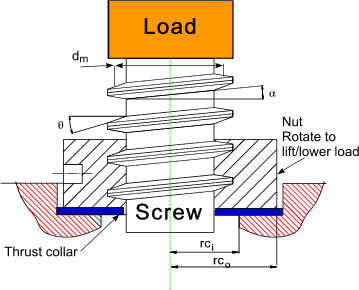Consider a Force F applied at a mean radius rm which causes the load to be raised. The nut is turning the screw is prevented from turning.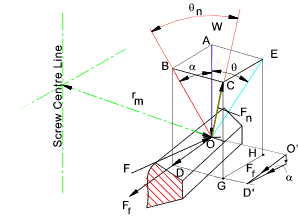The sketch above identifies the reactive forces acting at point O on the screw thread surface.
The reactive force Fn acting normal to the surface has the following components in the plane of interest ABDO.

 OD = Ff which is the friction force opposing movement up the thread surface( Ff = μs Fn ) OA = Is equal and opposite to the force being lifted. (W) OB = Is the vector sum of OD and OA and forms an angle θn with vector (OB = Fn cos θ n )

The sketch below illustrates the horizontal and vertical forces acting at a representative point at a radius r m in the plane normal to the radius.
For equilibrium the sum of all vertical forces = 0 and the sum of all horizontal forces = 0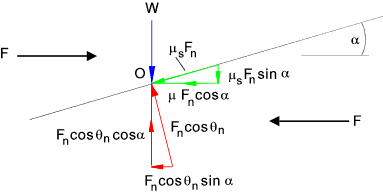Summing the forces in the vertical direction results in.

Fn cos θncos α = W + Ff sin α

The coefficient of friction for the screw surface materials is μs   : Ff = μs.Fn and therefore.

Fn = W / ( cosθncos α - μs.sin α )..........Equation A

Summing the moment of the forces around the centerline of the screw to obtain TR , the torque to raise the load W up the incline of the screw.

T R   =   F.r m   =   r m.(F f cos α + F n cos θnsin α )    =  r m.(μs.F n. cos α + F n cos θnsin α )

There is an additional friction torque resulting from the friction force on the thrust collar see top sketch above.  This friction force = μc. W. ( μc = coefficient of friction between the screw thrust surface and the collar surface.).  This friction torque is assumed to be acting at the thrust collar mean radius rmc

The total torque required to raise the load W is therefore equal to

T R   =  r m.(μ s.F n cos α + F n cos θnsin α ) + rmc.μc. W

Substituting for Fn.. see equation A above and replacing rm by dm/2 ..( and rmc by dmc /2 )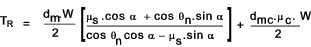dividing the first term numerator and denominator by cos α results in..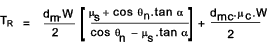.........Equation B

Tr = the torque in Nm to lift the load W (N)//

BC = AE = OA tan θ = (OB cosα). tanθ ..therefore
tan θn = BC/OB = cos α. tan θ ..therefore
θn = tan-1 [ cos α. tan θ]

For many applications the helix angle is small compared to the thread angle and therefore cos α is approximately equal to 1. e.g. For M20 2.5 pitch the value of cos α = 0.999

Therefore it is reasonable to let tan θn = tan θ and therefore θn = θ. ..
[However for multi start screws or screws with a relatively course lead (pitch) it is necessary to use θn ]

For normal screws and fine pitch power screws the above equation for TR can be written as :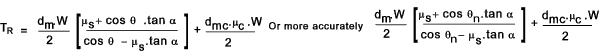The torque to lower the load is written as follows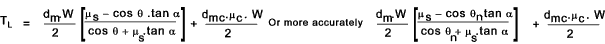These equations can be expressed in terms of the lead by substituting the relationship tan α = l / (θ.dm )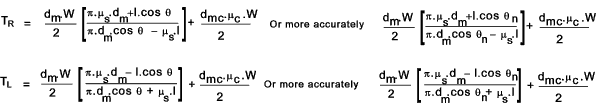For applications where the thrust is taken on ball or roller thrust bearing the value of μc is sufficiently low that it can be taken as approximately 0 and therefore the second term can be ignored.    The approximate equations reduce to..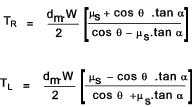Overhauling

Overhauling occurs when the screw helix angle is such that the load W would cause to screw to rotate when the rotating force F = zero i.e. the Force is not only required to raise the load - it is also required to statically support the load .

The overhauling torque To as calculated below will cause the screw to overhaul when To is less than zero.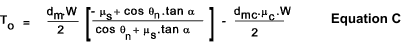If the thrust collar torque is assumed to be near zero then the helix angle which allows overhauling (To = < zero) can be solved.

tan α = < μs / cos θn

Screw Efficiency

The efficiency of a screw thread (Forward-Raising)can be defined as follows

η = Torque to raise load (zero friction) / Torque to raise load

Using equation B above the value of TR resulting when μs = 0 =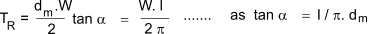Dividing this by equation B to provides an equation for the efficiency of the power screw thread.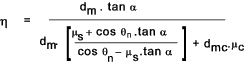If the collar friction is very low compared to the screw friction the equation reduces to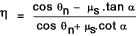Values of μs and μc are found on the friction coefficients page of this website Power screw friction factors

The efficiency of a screw thread (Backdriving -Lowering)can be defined as follows

η = Back torque generated /Back Torque generated (zero friction)

Using equation C above the value of TR resulting when μs = 0 =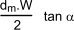Dividing equation C by this equation provides an equation for the efficiency of the power screw thread.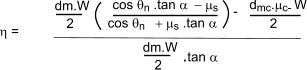If the collar friction is very low compared to the screw friction the equation reduces to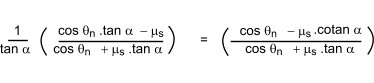Values of μs and μc are found on the friction coefficients page of this website Power screw friction factors

 Links to Power Screw Design Module 6 Power Screws ... Document download providing detailed notes and power screw equations. roton formulas ...Power screw equation simple equations Power screw calculation ... Useful ABC calculator
Machine_Parts_Index
Power screw Page